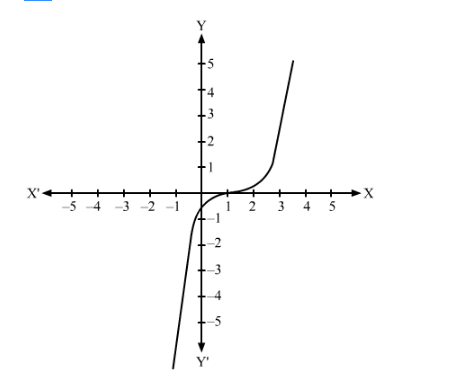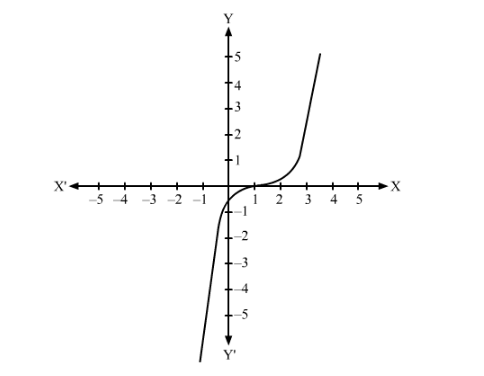# In Fig. 1, the graph of a polynomial p (x) is shown.`
Question:

In Fig. 1, the graph of a polynomial p (x) is shown. The number of zeroes of p (x) is

(a) 4

(b) 1

(c) 2

(d) 3Solution:

In the following graph we observe that it intersects x-axis at x = 1. So it has only one zero.Hence the correct option is (b)